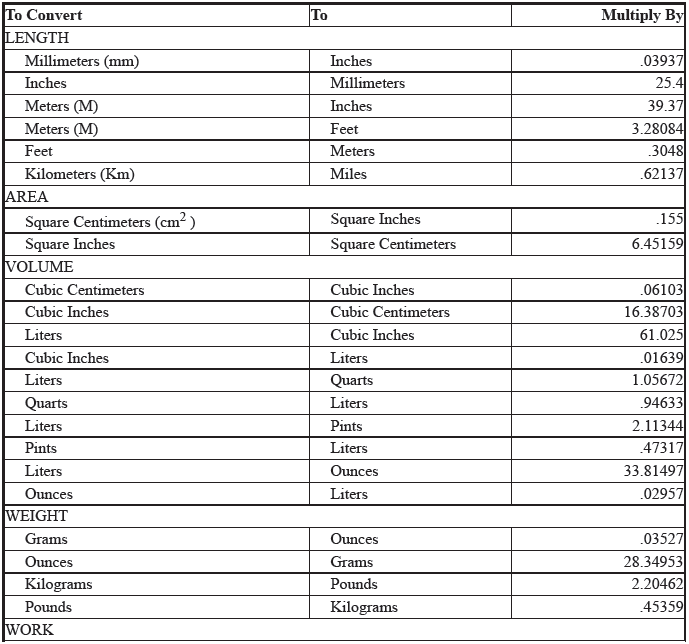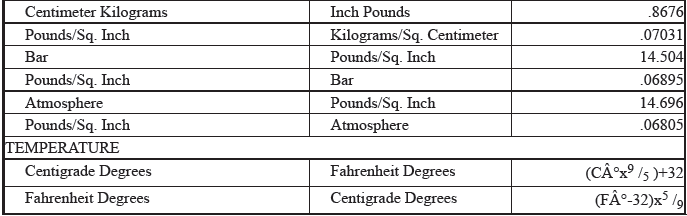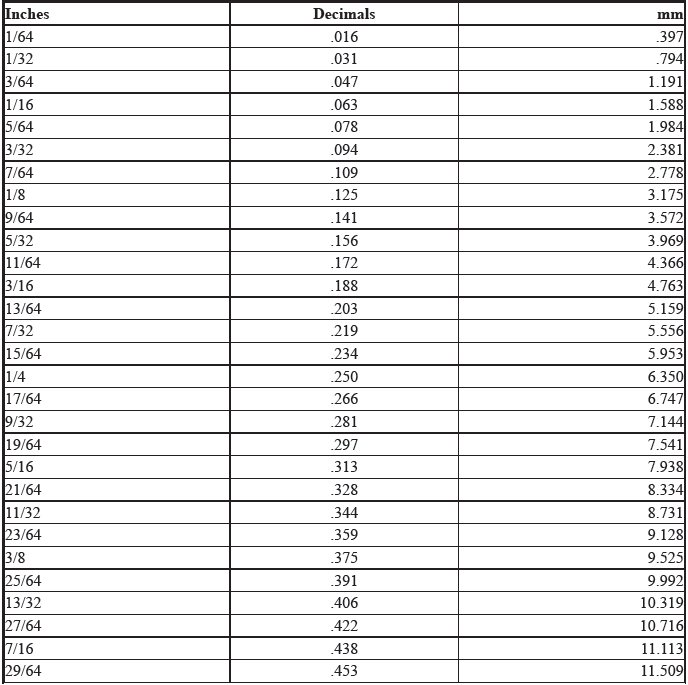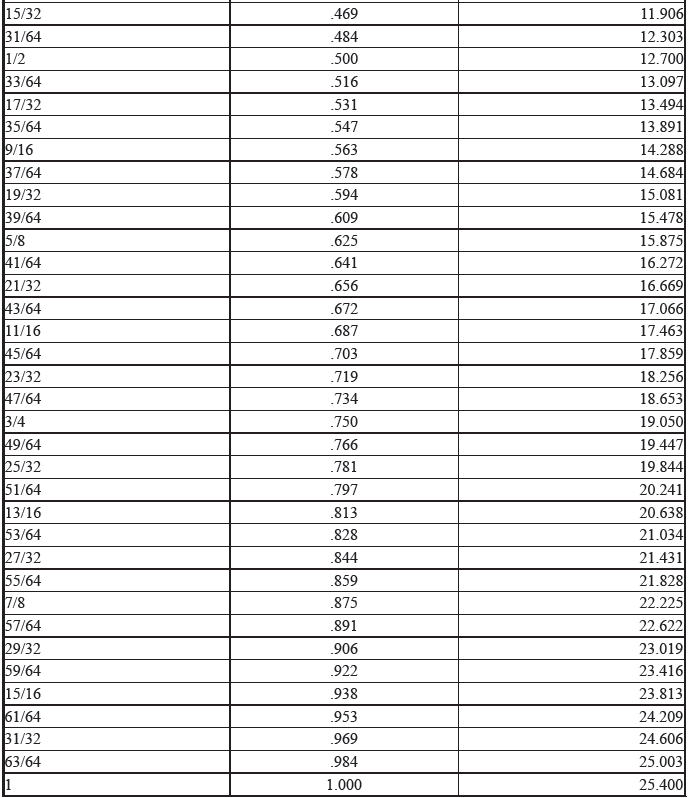# Honda HR-V: English-Metric Conversion Chart

Honda HR-V (2015-2021) Service Manual / General Information / English-Metric Conversion Chart

METRIC CONVERSIONS

Metric conversions are making life more difficult for the mechanic. In addition to increasing the number of tools required, metric-dimensioned nuts and bolts are used alongside English components in many new vehicles. The mechanic has to decide which tool to use, slowing down the job. The tool problem can be solved by trial and error, but some metric conversions aren't so simple.

Converting temperature, lengths or volumes requires a calculator and conversion charts, or else a very nimble mind. Conversion charts are only part of the answer though, because they don't help you "think" metric, or "visualize" what you are converting. The following examples are intended to help you "see" metric sizes:

LENGTH

Meters are the standard unit of length in the metric system. The smaller units are 10ths (decimeter), 100ths (centimeter), and 1000ths (millimeter) of a meter. These common examples might help you to visualize the metric units:

• A meter is slightly longer than a yard (about 40 inches).
• An aspirin tablet is about one centimeter across (.4 inches).
• A millimeter is about the thickness of a dime.

VOLUME

The metric weight system is based on the gram, with the most common unit being the kilogram (1000 grams).

Our comparable units are ounces and pounds:

• A kilogram is about 2.2 pounds.
• A kilogram is about 2.2 pounds.

TORQUE

Torque is somewhat complicated. The term describes the amount of effort exerted to turn something. A chosen unit of weight or force is applied to a lever of standard length. The resulting leverage is called torque. In our standard system, we use the weight of one pound applied to a lever a foot long, resulting in the unit called a foot-pound. A smaller unit is the inch-pound (the lever is one inch long). Metric units include the meter kilogram (lever one meter long with a kilogram of weight applied) and the Newton-meter (lever one meter long with force of one Newton applied). Some conversions are:

• A meter kilogram is about 7.2 foot pounds.
• A foot pound is about 1.4 Newton-meters.
• A centimeter kilogram (cmkg) is equal to.9 inch pounds.

PRESSURE

Pressure is another complicated measurement. Pressure is described as a force or weight applied to a given area.

Our common unit is pounds per square inch. Metric units can be expressed in several ways. One is the kilogram per square centimeter (kg/cm2). Another unit of pressure is the Pascal (force of one Newton on an area of one square meter), which equals about 4 ounces on a square yard. Since this is a very small amount of pressure, we usually see the kiloPascal, or kPa (1000 Pascals). Another common automotive term for pressure is the bar (used by German manufacturers), which equals 10 Pascals. Thoroughly confused? Try the examples below:

• Atmospheric pressure at sea level is about 14.7 psi.
• Atmospheric pressure at sea level is about 1 bar.
• Atmospheric pressure at sea level is about 1 kg/cm2.
• One pound per square inch is about 7 kPa.

CONVERSION FACTORS

CONVERSION FORMULAEQUIVALENCIES###DTC B0070-12